# Prediction With Expert Advice For The Brier Game

The paper gives a new algorithm for a natural probability forecasting problem and shows a tight performance bound. It also describes results of extensive experiments performed on novel data sets. The paper introduces a new algorithm and analysis for online prediction from expert advice for multi-class classification with square-loss.

A game of prediction consists of three components: the space of events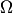, the space of predictions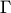, and the loss function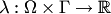which measures the quality of predictions. In our experiments we are interested in the "Brier game" (Brier, 1950), since it is widely used in probability forecasting.

Letbe a finite and non-empty set,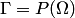be the set of all probability measures on, and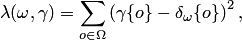where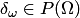is a probability measure concentrated at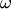: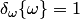and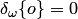for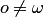.

The theoretical bound for its cumulative loss at a prediction step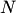isfor any expert, where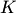is the number of experts. It is proved that the theoretical bound for the AA is optimal. It is also proved that the Brier game is mixable for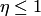. The substitution function for the algorithm is capable to give proper prediction even if the weights are not normalized at each step.

Experiments on the prediction of the result of football and tennis matches are made and show the theoretical bound is rather tight empirically. Bookmakers are used as experts, and the loss of the prediction algorithm is close to the loss of the best bookmaker.

The journal version of the paper describes the experiments with Khutsishvili's formula and makes a comparison with other prediction algorithms.

The Matlab package of the algorithms used is provided for public use.